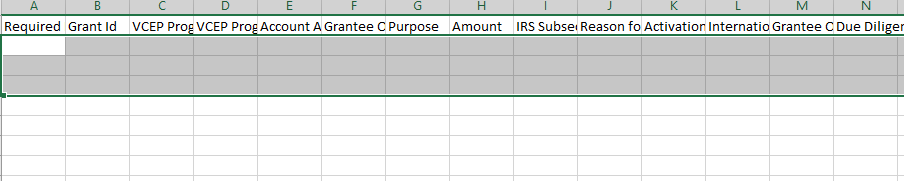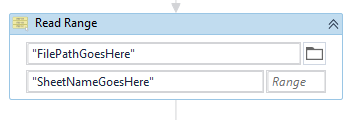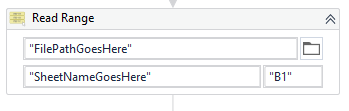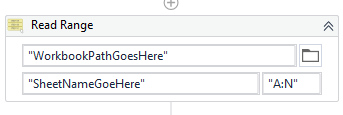# Copy Value from one excel to other excel without using for each loop

Excel 1:

Excel 2:How Can Copy the Value from excel 1 to Excel 2 with out using for each loop.

Copy Value from Column A:H and Column N (Excel 1) and ignore the column values I,J,K,L,M.

Note=> Copy the Rows are dynamic not fixed rows so how can we copy the values from Excel 1 to Excel 2, How to use read range excel activity for above Copy Value from A:H and Column N in Excel 1.

If the schema is same, then you can leave the Read Range range Empty while reading data from the first Excel Workbook, and it will copy the entire Range.Its not necessary to pass in the entire range, or if you wish to start from the second cell onwards, just pass in B1.Then you can simply use a Write Range to write that Table to the second Excel Workbook.

However, if you want to select specific column ranges, then let me know, I can devise a solution for you.

Kind Regards,
Ashwin A.K

I need specific column name like A:H AND N column need copy paste Excel 1 into excel 2, remaining column values need to be ignore from Excel1.

Could you give this workflow(link towards the bottom) a try and let me know if it works out for you?

First, we will provide the entire range i.e., A:N.I understand that you want A:H and then N, but we will take care of that by constructing an array of column names and use the DefaultView method to fetch the columns of interest.

This bit of code will fetch the entire set of column names for us.

``````dt.Columns.Cast(Of DataColumn).Select(Function(s) s.ColumnName).ToArray()
``````

This bit will convert the column name into an index we can will use for fetching the required columns.

``````UiPath.Excel.Helpers.ExcelUtilities.ConvertColumnLetterToColumnIndex("N")
``````

This is what will enable us to get the columns we require:

``````arr_columns.TakeWhile(Function(tk,i) i<int_midIndex).Append(arr_columns.Last()).ToArray()
``````

And finally, we will use the DefaultView to remove unwanted columns:

``````dt.DefaultView.ToTable(False,arr_reqColumns)
``````

CopySelectColumnsFromExcel.xaml (7.2 KB)

Kind Regards,
Ashwin A.K

Hello @sankar.mayilsamy ,

Have a look here for practicle implementation

Thanks,
Sanjit

There’s no reason to avoid For Each. Stop making things harder on yourself for no reason, use the activities that are designed for these things. Read Range, For Each with Write Range/Cell etc.